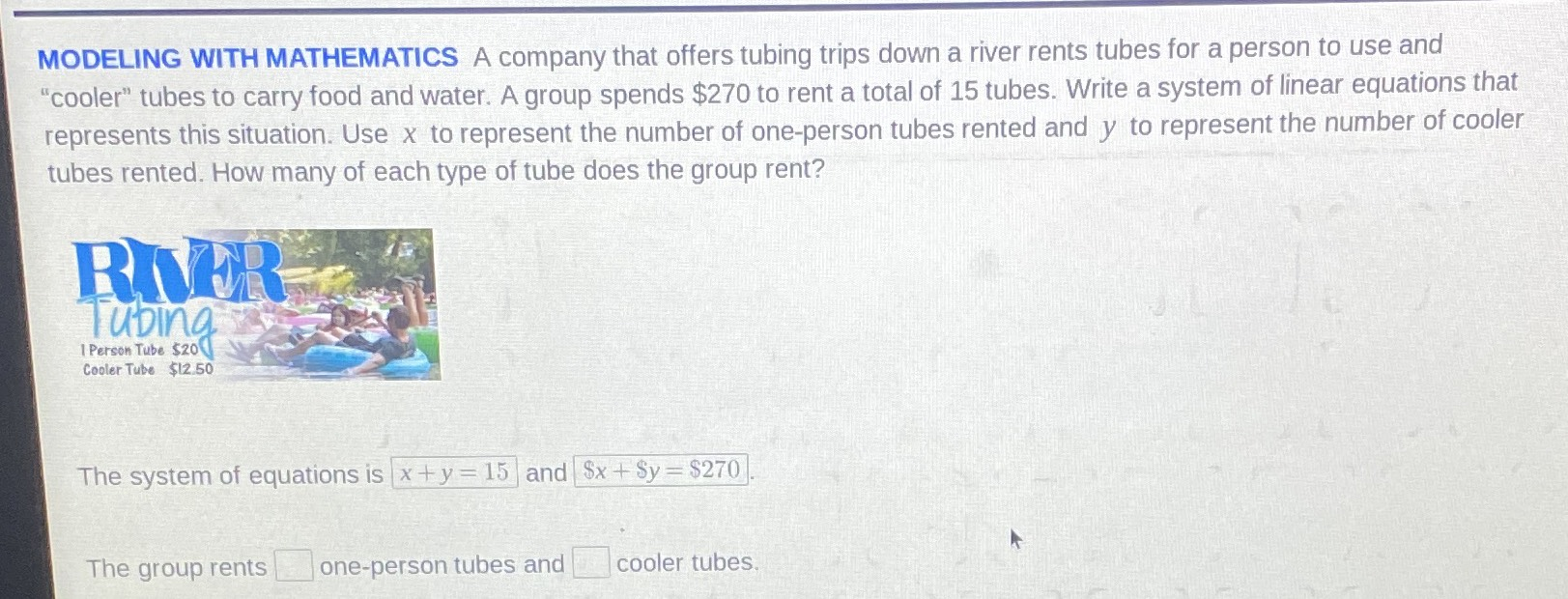### ¿Todavía tienes preguntas de matemáticas?

Pregunte a nuestros tutores expertos
Algebra
PreguntaA company that offers tubing trips down a river rents tubes for a person to use and "cooler" tubes to carry food and water. A group spends $$\ 270$$ to rent a total of $$15$$ tubes. Write a system of linear equations that represents this situation. Use $$x$$ to represent the number of one-person tubes rented and $$y$$ to represent the number of cooler tubes rented. How many of each type of tube does the group rent?

The system of equations is $$x + y = 15$$ and $$\ x + \ y = \ 270$$

The group rents $$\square$$ one-person tubes and $$\square$$ cooler tubes.

$$x+ y= 15\\20x+ 12.5y= 270\\x= 11,y= 4$$